SSC  >  SSC CPO Mock Test (Quant Apt) 6

# SSC CPO Mock Test (Quant Apt) 6

Test Description

## 50 Questions MCQ Test SSC CPO & Constable - Mock Tests & Previous Year Papers | SSC CPO Mock Test (Quant Apt) 6

SSC CPO Mock Test (Quant Apt) 6 for SSC 2023 is part of SSC CPO & Constable - Mock Tests & Previous Year Papers preparation. The SSC CPO Mock Test (Quant Apt) 6 questions and answers have been prepared according to the SSC exam syllabus.The SSC CPO Mock Test (Quant Apt) 6 MCQs are made for SSC 2023 Exam. Find important definitions, questions, notes, meanings, examples, exercises, MCQs and online tests for SSC CPO Mock Test (Quant Apt) 6 below.
Solutions of SSC CPO Mock Test (Quant Apt) 6 questions in English are available as part of our SSC CPO & Constable - Mock Tests & Previous Year Papers for SSC & SSC CPO Mock Test (Quant Apt) 6 solutions in Hindi for SSC CPO & Constable - Mock Tests & Previous Year Papers course. Download more important topics, notes, lectures and mock test series for SSC Exam by signing up for free. Attempt SSC CPO Mock Test (Quant Apt) 6 | 50 questions in 30 minutes | Mock test for SSC preparation | Free important questions MCQ to study SSC CPO & Constable - Mock Tests & Previous Year Papers for SSC Exam | Download free PDF with solutions
 1 Crore+ students have signed up on EduRev. Have you?
SSC CPO Mock Test (Quant Apt) 6 - Question 1

### The ratio between the ages of P and Q four yrs ago was 5 : 6. If the total of their ages at present is 52 yrs, what is the ratio of their present ages?

Detailed Solution for SSC CPO Mock Test (Quant Apt) 6 - Question 1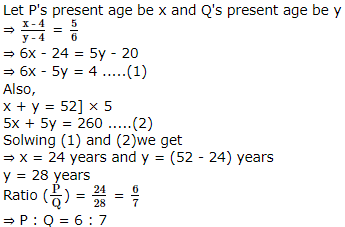SSC CPO Mock Test (Quant Apt) 6 - Question 2

### If x + y = 6 and 3x - y = 4, then x - y is equal to

Detailed Solution for SSC CPO Mock Test (Quant Apt) 6 - Question 2

x + y = 6
⇒ y = 6 - x
Now, 3x - y = 4
or 3x - (6 - x) = 4
or 3x - 6 + x = 4
or 4x = 10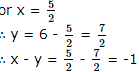SSC CPO Mock Test (Quant Apt) 6 - Question 3

### A train starts with full of passenges.At the first station,the train drops one-third of the passengers and takes in 96 more.At the next station,one half of the passengers on board get down while 12 new passengers get on board.The number of passengers now is 240.The number of passengers in the beginning was

Detailed Solution for SSC CPO Mock Test (Quant Apt) 6 - Question 3

Let the number of passengers be x when the train started off
∴ At the first station, the number of passengers = x - x/3 + 96 = 2x/3 + 96
At the second station, the number of passengers = ( 2x/3 + 96) 1/2 + 12
At the third station, the number of passengers left = x/3 + 48 + 12 = 240
⇒ x/3 = 180
⇒ x = 540

SSC CPO Mock Test (Quant Apt) 6 - Question 4
If (2x+3) and (x-1) are two factors of (2x2 + x)2 - 4(2x2 + x) + 3, then the remaining two factors are
Detailed Solution for SSC CPO Mock Test (Quant Apt) 6 - Question 4 (2x2 + x)2 - 4(2x2 + x) + 3
= 4x4 + x2 + 4x3 - 8x2 - 4x + 3
= 4x4 + 4x3 - 7x2 - 4x + 3
= 4x3 (x - 1) + 8x2 (x - 1) + x (x - 1) - 3 (x - 1)
= (x - 1) (4x3 + 8x2 + x - 3)
= (x - 1) [2x2 (2x + 3) + x(2x + 3) - 1(2x + 3)]
= (x - 1) (2x + 3) (2x2 + x - 1)
= (x - 1) (2x + 3) [2x2 + 2x - x - 1]
= (x - 1) (2x + 3) [(2x (x + 1)) - 1(x + 1)]
= (x - 1) (2x + 3) (x + 1) (2x - 1)
SSC CPO Mock Test (Quant Apt) 6 - Question 5
Total number of boys and girls eligible for the book grant is 9. Each boy is given a grant of Rs 25 and each girl Rs 20. If the total amount given as grant is Rs 200,the number of girls eligible for grant is
SSC CPO Mock Test (Quant Apt) 6 - Question 6

A company produces on an average 4000 items per month for the first 3 months. How many items it must produce on an average per month over the next 9 months, to average 4375 items per month over the whole?

Detailed Solution for SSC CPO Mock Test (Quant Apt) 6 - Question 6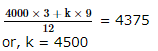∴ 4500 items per month should be produced for the last 9 months

SSC CPO Mock Test (Quant Apt) 6 - Question 7

Diagonals of a rhombus are in the ratio 3:4 and its area is 2400 cm2. Side of the rhombus is

Detailed Solution for SSC CPO Mock Test (Quant Apt) 6 - Question 7

Let the diagonals of a rhombus are 3K and 4K. Then
Area of rhombus = 1/2 x product of diagonals
2400 = 1/2 x 3K x 4K
or 400 = K2
or K = 200
∴ The Diagonals are 60 cm and 80 cm
we know that, if a be side of the rhombus and AC and BD be its diagonals then
(AC)2 + (BD)2 = 4 x a2
or (60)2 + (80)2 = 4 x a2
or 3600 + 6400 = 4 x a2
or 10000 = 4a2
or 2500 = a2
or a = 50 cm

SSC CPO Mock Test (Quant Apt) 6 - Question 8

A rectangular park is 200 by 150m. Two cross paths are to be made one parallel to the length and the other parallel to the breadth. The longer path is to be 3 m wide and the shorter is to be 2 m wide. The cost of grassing the remaining part of the park @ Rs. 1000 per ha is

Detailed Solution for SSC CPO Mock Test (Quant Apt) 6 - Question 8

Area of the park minus the paths
= (200 - 3) (150 - 2)
= 197 × 147 sq. m
= 29156 sq. m
The cost of grashing the remaming part of the park = Rs 29156/10000 x 1000
= Rs 2915.6

SSC CPO Mock Test (Quant Apt) 6 - Question 9

The area of the sector of the circle is 1/10th the area of the circle. The angle of the sector is​

Detailed Solution for SSC CPO Mock Test (Quant Apt) 6 - Question 9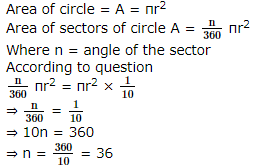SSC CPO Mock Test (Quant Apt) 6 - Question 10

The average of 50 numbers is 30. If two numbers, 35 ad 40 are discarded, then the average of the remaining numbers is nearly:

Detailed Solution for SSC CPO Mock Test (Quant Apt) 6 - Question 10

Sum of 50 number = 30 x 50 = 1,500
Sum of remaining 48 numbers = 1,500 - (35 + 40) = 1,425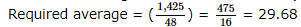SSC CPO Mock Test (Quant Apt) 6 - Question 11

If the equation 12x² + 7xy - py² - 18x + y + 6 = 0 represents a pair of perpendicular lines, then

SSC CPO Mock Test (Quant Apt) 6 - Question 12
At what per cent annum will Rs 3000 amount to Rs 3993 in 3 years if the interest is compounded annually?
SSC CPO Mock Test (Quant Apt) 6 - Question 13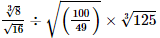is equal to

SSC CPO Mock Test (Quant Apt) 6 - Question 14
The marked price of a watch was Rs 720. A man bought the same for Rs 550.80 after getting two successive discounts,the first being 10%. What was the second discount rate?
SSC CPO Mock Test (Quant Apt) 6 - Question 15
Two-third of a positive number and 25/216 of its reciprocal are equal. The number is
SSC CPO Mock Test (Quant Apt) 6 - Question 16

AB∥CD and P is a point between the lines, the measure of the angles ∠ABP+∠BPD+∠CDP is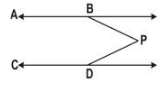SSC CPO Mock Test (Quant Apt) 6 - Question 17
Statement-"A curve is always a locus but a locus need not be a curve." The statement is
SSC CPO Mock Test (Quant Apt) 6 - Question 18
Radius of a circle is 61 cm. If a chord of a this circle is 11 cm from the centre then the length of the chord is
Detailed Solution for SSC CPO Mock Test (Quant Apt) 6 - Question 18 Using Pythograrous theorem as in above MB = 60 cm so length of chord = 120 cm.
SSC CPO Mock Test (Quant Apt) 6 - Question 19

Lines AB and CD intersect at O. This forms four angles. If sum of three of these is 299° then the measure of the fourth angle is

Detailed Solution for SSC CPO Mock Test (Quant Apt) 6 - Question 19

Sum of all angles about a point is 360
299 + x = 360°
so, x = 61

SSC CPO Mock Test (Quant Apt) 6 - Question 20
Find the least multiple of 23,which when divided by 18,21 and 24 leaves the remainders 7,10 and 13 respectively.
SSC CPO Mock Test (Quant Apt) 6 - Question 21

A light house is 60 metres high with its base being at the sea level.If the angle of depression of a boat in the sea from the top of the light house is  15°,then the distance of the boat from the foot of the light house is equal to

SSC CPO Mock Test (Quant Apt) 6 - Question 22
The sum of the two digits number is 15. If 9 be added to the number, then the digits are reversed. The number is
SSC CPO Mock Test (Quant Apt) 6 - Question 23
A district has 64000 inhabitants. If the population increases at the rate of 2(1/2)% per annum, then the number of inhabitants at the end of 3 years will be
SSC CPO Mock Test (Quant Apt) 6 - Question 24
In a school 70% of the students are girls. The number of boys is 510. Then the total number of students in the school is
SSC CPO Mock Test (Quant Apt) 6 - Question 25

A tap can fill a tank in one hour. A second tap empty it in 30 minutes. If both the taps operate simultaneously, how much time is needed to empty the tank?

Detailed Solution for SSC CPO Mock Test (Quant Apt) 6 - Question 25

In one minute 1/60 + 1/30 = 1/20 of the tank will be empty

SSC CPO Mock Test (Quant Apt) 6 - Question 26
A book-seller bought 200 textbooks for Rs 12,000. He wanted to sell them at a profit so that he got 20 books free. At what profit percent should he sell them?
SSC CPO Mock Test (Quant Apt) 6 - Question 27

Kewal sells two tape recorders at the same price. On one,he gains 10% and on the other, he loses 10%. The total gain or loss in the transaction is

Detailed Solution for SSC CPO Mock Test (Quant Apt) 6 - Question 27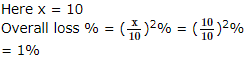SSC CPO Mock Test (Quant Apt) 6 - Question 28

In a triangle AC, if a = 2, B = 60° and c = 75°, then b =

SSC CPO Mock Test (Quant Apt) 6 - Question 29
The ratio between the present ages of P and Q is 3:4 respectively. If P's age is 20 yrs,what will be the ratio of their ages after 5 yrs?
SSC CPO Mock Test (Quant Apt) 6 - Question 30

Rs 6400 are divided among three workers in the ratio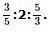. The share of the second worker (in rupees)is

Detailed Solution for SSC CPO Mock Test (Quant Apt) 6 - Question 30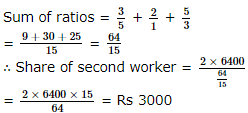SSC CPO Mock Test (Quant Apt) 6 - Question 31
If 4th term of an H.P. is 5 and 5th term is 4, then its 20th term is
SSC CPO Mock Test (Quant Apt) 6 - Question 32

The interest on Rs 4500 from January 5 to May 31, 1954 at 8% is

Detailed Solution for SSC CPO Mock Test (Quant Apt) 6 - Question 32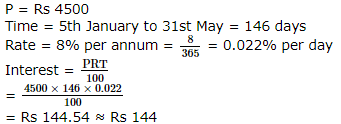SSC CPO Mock Test (Quant Apt) 6 - Question 33

The square root of 0.09 + 2x0.21 + 0.49 is

SSC CPO Mock Test (Quant Apt) 6 - Question 34

A person travels equal distances with speeds of 3 km/hr, 4 km/hr and 5 km/hr and takes a total time of 47 minutes. The total distance (in km) is:

Detailed Solution for SSC CPO Mock Test (Quant Apt) 6 - Question 34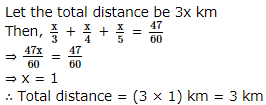SSC CPO Mock Test (Quant Apt) 6 - Question 35
12 women complete a work in 20 days. If only 8 women are employed, the time required to complete the same work is
SSC CPO Mock Test (Quant Apt) 6 - Question 36

cotx - tanx =

Detailed Solution for SSC CPO Mock Test (Quant Apt) 6 - Question 36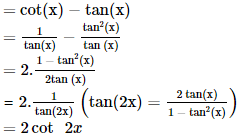SSC CPO Mock Test (Quant Apt) 6 - Question 37

sin 20° sin 40° sin 60° sin 80°=

SSC CPO Mock Test (Quant Apt) 6 - Question 38

[(sinθ+sin2θ)/(1+cosθ+cos2θ)]=

SSC CPO Mock Test (Quant Apt) 6 - Question 39

A solid metallic spherical ball of diameter 6 cm is melted and recast into a cone with diameter of the base as 12 cm. The height of the cone is :

Detailed Solution for SSC CPO Mock Test (Quant Apt) 6 - Question 39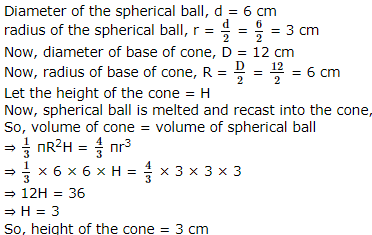SSC CPO Mock Test (Quant Apt) 6 - Question 40

A cube of edges 5 cm is cut into cubes each of edge 1 cm. The ratio of the total surface area of one of the small cubes to that of the large cube is equal to :

Detailed Solution for SSC CPO Mock Test (Quant Apt) 6 - Question 40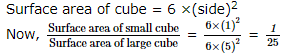SSC CPO Mock Test (Quant Apt) 6 - Question 41
The radii of two right circular cylinder are in ratio 2: 3 and their heights are in ratio 5:4. The ratio of their curved surface areas is
SSC CPO Mock Test (Quant Apt) 6 - Question 42

Study the following graph showing the sales forecast for the next ten weeks, to answer these questions.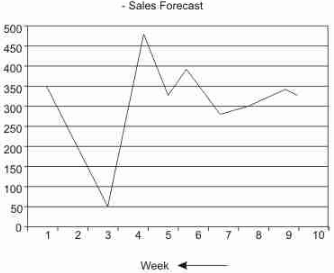Q. If the forecasted demand is met by having uniform production during the weeks at an average level, then the number of weeks during which demand will not be met is :

Detailed Solution for SSC CPO Mock Test (Quant Apt) 6 - Question 42

Average sales forecast for 10 weeks = 322
Hence there are 4 weeks during which demand will not be met

SSC CPO Mock Test (Quant Apt) 6 - Question 43

Study the following graph showing the sales forecast for the next ten weeks, to answer these questions.Q. If the production is uniform, then what should be the minimum capacity of the storage space to store the units in excess of demand?.

SSC CPO Mock Test (Quant Apt) 6 - Question 44

Study the following graph showing the sales forecast for the next ten weeks, to answer these questions.Q. If the maximum production capacity is 300 units, then the demand that will not be met will be

Detailed Solution for SSC CPO Mock Test (Quant Apt) 6 - Question 44

unmet demand = 475 - 300 = 175

SSC CPO Mock Test (Quant Apt) 6 - Question 45

Study the following graph showing the sales forecast for the next ten weeks, to answer these questions.Q. During which year was the percentage rise/fall in sales from the previous year the higest for company A ?

SSC CPO Mock Test (Quant Apt) 6 - Question 46

The following questions are based on the following pie chart which gives the expenditure incurred in printing a magazine.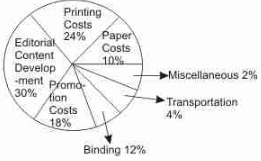Q. What is the angle for the sector representing paper cost ?

Detailed Solution for SSC CPO Mock Test (Quant Apt) 6 - Question 46

Angle for sector representing paper cost = 360/100 × 10 = 36°

SSC CPO Mock Test (Quant Apt) 6 - Question 47

The following questions are based on the following pie chart which gives the expenditure incurred in printing a magazine.Q. What should be the central angle of the sector representing transportation charges ?

Detailed Solution for SSC CPO Mock Test (Quant Apt) 6 - Question 47

Angle for sector representing transportation = 360/100× 4 = 14.4°

SSC CPO Mock Test (Quant Apt) 6 - Question 48

The following questions are based on the following pie chart which gives the expenditure incurred in printing a magazine.Q. If the editorial contents development cost is Rs.30,000, then the cost of transportation can be expected to be

Detailed Solution for SSC CPO Mock Test (Quant Apt) 6 - Question 48

30% = 30,000
1% = 1000
∴ 4% = Rs 4000

SSC CPO Mock Test (Quant Apt) 6 - Question 49

The following questions are based on the following pie chart which gives the expenditure incurred in printing a magazine.Q. For a given issue of the magazine , the miscellaneous cost is Rs.2000 and the print-run is 12500 copies. What should be the sale price if the publisher desires a profit of 5% ?

Detailed Solution for SSC CPO Mock Test (Quant Apt) 6 - Question 49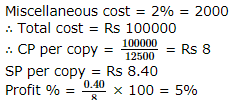SSC CPO Mock Test (Quant Apt) 6 - Question 50

The following questions are based on the following pie chart which gives the expenditure incurred in printing a magazine.Q. If for the same data as given in the previous question , the print-run were to be 50000 copies , the sale price per copy would have been :

Detailed Solution for SSC CPO Mock Test (Quant Apt) 6 - Question 50

Cost price per copy = 100000/50000 = Rs 2
SP at a profit of 5%
SP = 2 × 1.05 = Rs 2.10

## SSC CPO & Constable - Mock Tests & Previous Year Papers

1 docs|63 tests
 Use Code STAYHOME200 and get INR 200 additional OFF Use Coupon Code
Information about SSC CPO Mock Test (Quant Apt) 6 Page
In this test you can find the Exam questions for SSC CPO Mock Test (Quant Apt) 6 solved & explained in the simplest way possible. Besides giving Questions and answers for SSC CPO Mock Test (Quant Apt) 6, EduRev gives you an ample number of Online tests for practice

1 docs|63 tests